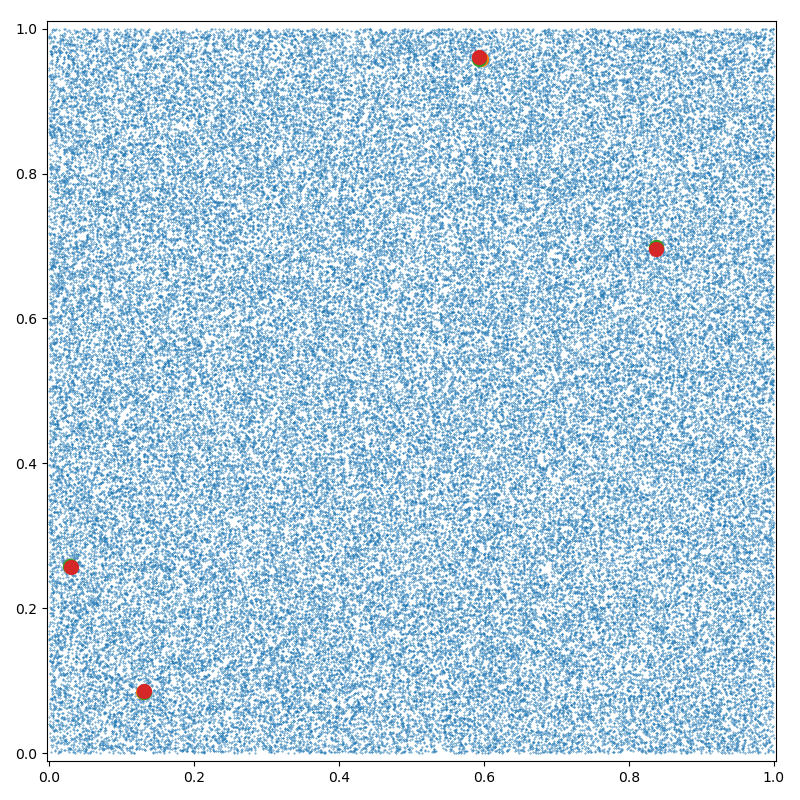Arg-K-Min reduction¶

Using the pykeops.numpy API, we define a dataset of N points in $$\mathbb R^D$$ and compute for each point the indices of its K nearest neighbours (including itself).

Setup¶

Standard imports:

import time

import matplotlib.pyplot as plt
import numpy as np

from pykeops.numpy import Genred

Define our dataset:

N = 100000  # Number of points
D = 2  # Dimension of the ambient space
K = 3  # Number of neighbors to look for

dtype = "float32"  # May be 'float32' or 'float64'

x = np.random.rand(N, D).astype(dtype)

KeOps Kernel¶

formula = "SqDist(x,y)"  # Use a simple Euclidean (squared) norm
variables = [
"x = Vi(" + str(D) + ")",  # First arg : i-variable, of size D
"y = Vj(" + str(D) + ")",
]  # Second arg: j-variable, of size D

# N.B.: The number K is specified as an optional argument opt_arg
my_routine = Genred(
formula, variables, reduction_op="ArgKMin", axis=1, dtype=dtype, opt_arg=K
)

Using our new pykeops.numpy.Genred routine, we perform a K-nearest neighbor search ( reduction_op = "ArgKMin" ) over the $$j$$ variable $$y_j$$ ( axis = 1):

Note

If CUDA is available and backend is "auto" or not specified, KeOps will:

1. Load the data on the GPU

2. Perform the computation on the device

3. Unload the result back to the CPU

as it is assumed to be most efficient for large-scale problems. By specifying backend = "CPU" in the call to my_routine, you can bypass this procedure and use a simple C++ for loop instead.

# Dummy first call to warm-up the GPU and thus get an accurate timing:
my_routine(np.random.rand(10, D).astype(dtype), np.random.rand(10, D).astype(dtype))

# Actually perform our K-nn search:
start = time.time()
ind = my_routine(x, x, backend="auto")
print("Time to perform the K-nn search: ", round(time.time() - start, 5), "s")

# The result is now an (N,K) array of integers:
print("Output values :")
print(ind)

plt.figure(figsize=(8, 8))
plt.scatter(x[:, 0], x[:, 1], s=25 * 500 / len(x))

for k in range(K):  # Highlight some points and their nearest neighbors
plt.scatter(x[ind[:4, k], 0], x[ind[:4, k], 1], s=100)

plt.axis("equal")
plt.axis([0, 1, 0, 1])
plt.tight_layout()
plt.show()Out:

Time to perform the K-nn search:  0.02378 s
Output values :
[[    0 83742 53806]
[    1 56841 32298]
[    2   549 88204]
...
[99997 67651 43806]
[99998 14512  7927]
[99999  7856 40348]]

Total running time of the script: ( 0 minutes 0.377 seconds)

Gallery generated by Sphinx-Gallery